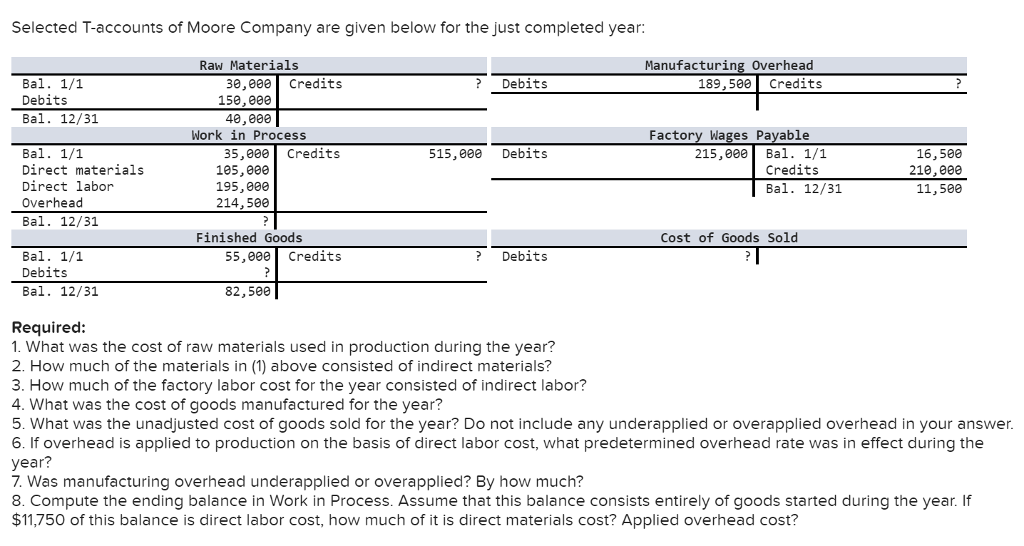# High quality homework help from top writers

Every paper is written from scratch. Our top homework writers will please you with excellent quality and timing for your paper# [SOLVED] Question & Answer: Selected T-accounts of Moore Company are given below for the just completed year: What was the cost ofSelected T-accounts of Moore Company are given below for the just completed year: What was the cost of raw materials used in production during the year? How much of the materials in (1) above consisted of indirect materials? How much of the factory labor cost for the year consisted of indirect labor? What was the cost of goods manufactured for the year? What was the unadjusted cost of goods sold for the year? Do not include any underapplied or overapplied overhead in your answer. If overhead is applied to production on the basis of direct labor cost, what predetermined overhead rate was in effect during the year? Was manufacturing overhead underapplied or overapplied? By how much? Compute the ending balance in Work in Process. Assume that this balance consists entirely of goods started during the year. If \$11,750 of this balance is direct labor cost, how much of it is direct materials cost? Applied overhead cost?

Don't use plagiarized sources. Get Your Custom Essay on
[SOLVED] Question & Answer: Selected T-accounts of Moore Company are given below for the just completed year: What was the cost of
From \$8/Page

Question – 1

Cost of material used in the production = Total available – Balance at the ending

= 30000 + 150000 – 40000 = 140000 worth of material used in production.

Question – 2

Work in process is debited with material cost of 105000 which is direct material. So Indirect material shall be 140000 – 105000 = 35000

Question – 3

Total Wager cost of current year = 210000 ( see in T-account – Factory wage cost)

Out of this Direct wage cost = 195000 ( refer to WIP account)

So Indirect wage cost = 210000 – 195000 = 15000

Question – 4

Cost of goods manufactured = 515,000 ( refer to WIP a/c …credits)

Question – 5

Cost of goods sold = Beginning Finished goods + Cost of goods manufactured – Ending Finished goods

= 55000 + 515000 – 82500 = 487,500

Question – 6

Predetermined rate based on direct wages = Factory Overhead applied to production / Direct wages * 100

= 214500 / 195000 * 100 = 110%

Question – 7

Under / over absorption of overhead

WIP account indactes that 214500 was absorbed during the year. But Factory overhead a/c indicates total debits of 189,500 ……..So this clearly over absorption.

Over absorption of overhead = 214500 – 189500 = 25000

Question – 8

Ending WIP = Total debits in WIP —– 549500 and credits = 515000. So balance = 34500

When wages are 11750, we have overhead = 11750 * 110% = 12925

So Material cost = 34500 – 11750 – 12925 = 9825